# Module 6: Odds and Ends

#### A Simple Exercise

In-Class Exercise 6.1: Download this template and solve the following problem. Suppose you are given a 2D array of integers and that each position in the array has the value 0 or 1. (Thus, a binary matrix). A sub-array of the matrix is considered a "chessboard" if the following properties hold: (1) the number of rows equals the number of columns (i.e. it is square); (2) there are at least two rows; (3) the 0's and 1's alternate as in a chessboard (i.e., none of the neighboring positions of a 0 has a 0). Your goal is to find the largest sub-matrix that is a chessboard. Analyse the complexity of your algorithm when the array size is N x N. Start by writing pseudocode.

#### Profiling

Most programming languages include supporting tools such as: (Java example)

• Debuggers (jdb).
• IDE's: Integrated Development Environments (Forte).
• Profilers (runhprof).

A profiler

• Inserts "hooks" into your code to enable periodic sampling.
• Builds an execution profile of your code:
=> how much time was spent in each method.

A Java profiling example:

• Consider the following sample program:
• We are given an array of numbers, randomly generated.
• Partial sum: sum of the first k numbers.
• Partial maximum: max of the first k numbers.
• Objective: what is the average partial sum (max) for a randomly chosen index?

• Here is some sample code: (source file)
```
public class ProfileTest {

static int numTrials;      // The number of trials to use in averaging.
static int size;           // Data (array) size.
static int[] data;         // The data.

// Find partial maximum: the max value in data,...,data[limit].

static int findMax (int limit)
{
int max = data;
for (int i=1; i < limit; i++)
if (data[i] > max)
max = data[i];
return max;
}

// Find partial sum: the sume of data,...,data[limit].

static double findSum (int limit)
{
double sum = 0;
for (int i=0; i < limit; i++)
sum += data[i];
return sum;
}

static void estimateMax()
{
// 1. Maintain total.
double total = 0;

// 2. Repeat numTrials times.
for (int n=0; n < numTrials; n++) {

// 2.1 Pick a sub-array randomly.
int limit = (int) UniformRandom.uniform (0, size-1);

// 2.2 Compute partial max.
int value = findMax (limit);

// 2.3 Accumulate.
total += value;
}

// 3. Compute average.
double avg = (double) total / (double) numTrials;

// 4. Output.
System.out.println ("Estimate of maximum=" + avg);
}

static void estimateSum()
{
// 1. Maintain total.
double total = 0;

// 2. Repeat numTrials times.
for (int n=0; n < numTrials; n++) {

// 2.1 Pick a sub-array randomly.
int limit = (int) UniformRandom.uniform (0, size-1);

// 2.2 Compute partial sum.
double value = findSum (limit);

// 2.3 Accumulate.
total += value;
}

// 3. Compute average.
double avg = (double) total / (double) numTrials;

// 4. Output.
System.out.println ("Estimate of sum=" + avg);
}

static void createData ()
{
// Draw the data randomly between 0 and "size":
data = new int [size];
for (int i=0; i < size; i++)
data[i] = (int) UniformRandom.uniform (1, size);
}

public static void main (String[] argv)
{
try {
// Command-line arguments contain the array size and number of trials.
if ( (argv == null) || (argv.length != 2) ) {
System.out.println ("Usage: java ProfileTest  ");
System.exit(1);
}

// Obtain the size of the data.
size = Integer.parseInt (argv.trim());

// Obtain the number of trials used in averaging.
numTrials = Integer.parseInt (argv.trim());

// Create random data.
createData ();

// Estimate the average partial-maximum.
estimateMax ();

// Estimate the average partial-sum.
estimateSum ();
}
catch (Exception e) {
e.printStackTrace();
}
}

}
```
• Notice that findMax is repeatedly called for the estimation.
• Also, findMax re-computes a partial maximum each time it is called.
• To execute on an array of size 1000 for an estimate with 100000 samples:
```
java ProfileTest 1000 100000
```

• Let's run a profile on this code:
• Profiling in Java is supported in the Java Virtual Machine (JVM).
• To use it, you compile a program normally but execute with "profiling on".
• Example:
```
java -Xrunhprof:cpu=samples,file=log.txt,depth=10 ProfileTest 1000 100000
```
• For details on the options, type
```
java -Xrunhprof:help
```
• The output file log.txt contains a sample of how much time was spent in various methods, e.g.,
```
CPU SAMPLES BEGIN (total = 37)
rank   self  accum   count trace method
1 32.43% 32.43%      12    15 ProfileTest.findSum
2 18.92% 51.35%       7    13 ProfileTest.findMax
3  5.41% 56.76%       2    19 java.lang.StrictMath.floor
4  5.41% 62.16%       2     3 java.util.jar.Manifest.parseName
5  5.41% 67.57%       2    18 UniformRandom.uniform
6  2.70% 70.27%       1     8 java.util.HashMap.put
7  2.70% 72.97%       1     7 java.lang.String.< init >
8  2.70% 75.68%       1     2 java.lang.Character.toLowerCase
9  2.70% 78.38%       1     9 java.util.jar.JarFile.getManifest
10  2.70% 81.08%       1    10 java.util.jar.Attributes.read
11  2.70% 83.78%       1     6 java.lang.Object.clone
12  2.70% 86.49%       1     4 java.util.jar.Attributes.< init >
13  2.70% 89.19%       1     1 java.util.jar.Manifest.parseName
14  2.70% 91.89%       1    14 java.lang.FloatingDecimal.dtoa
15  2.70% 94.59%       1     5 java.util.jar.Attributes.< init >
16  2.70% 97.30%       1    16 UniformRandom.uniform
17  2.70% 100.00%       1    17 java.lang.Math.floor
CPU SAMPLES END
```
Thus, the most time was spent in findSum, according to this estimate.

• The JVM provides an API for "profiling applications" to use in monitoring a program.
• runhprof is one such application.
• Currently (as of 2001), both the JVMPI and runhprof are not yet stable.

• Next, let's improve the code for only the maximum's:
• We'll leave the partial-sum computation as is, for comparison.
• Note: partial maxima can be computed once.
• Here's part of the code: (source file)
```
public class ProfileTest2 {

// ...

// findMax now returns the stored value.

static int findMax (int limit)
{
return max[limit];
}

// ...

static void estimateMax()
{
// 1. First find partial maximums.
max = new int [data.length];

for (int k=0; k < max.length; k++) {

max = data;
int m = data;

// 1.1 Find the partial maximum for each value of k.
for (int i=1; i<=k; i++)
if (data[i] > m)
m = data[i];

// 1.2 Store as k-th partial maximum.
max[k] = m;
}

// 2. Now estimate.
double total = 0;

// 3. Repeat numTrials times.
for (int n=0; n < numTrials; n++) {
// 3.1 Pick a sub-array randomly.
int limit = (int) UniformRandom.uniform (0, size-1);
// 3.2 Compute partial max.
int value = findMax (limit);
// 3.3 Accumulate.
total += value;
}

// 4. Compute average.
double avg = (double) total / (double) numTrials;

// 5. Output.
System.out.println ("Estimate of maximum=" + avg);
}

// ...

}
```
• This results in much less time computing the maxima (compared to sums):
```
CPU SAMPLES BEGIN (total = 28)
rank   self  accum   count trace method
1 32.14% 32.14%       9    18 ProfileTest2.findSum
2  7.14% 39.29%       2    12 ProfileTest2.estimateMax
3  7.14% 46.43%       2    15 UniformRandom.uniform
4  3.57% 50.00%       1     3 java.lang.Object.clone
5  3.57% 53.57%       1    16 UniformRandom.uniform
6  3.57% 57.14%       1    20 UniformRandom.uniform
7  3.57% 60.71%       1    14 java.lang.StrictMath.floor
8  3.57% 64.29%       1     7 java.lang.StringBuffer.append
9  3.57% 67.86%       1     1 java.util.jar.Manifest.parseName
10  3.57% 71.43%       1    13 UniformRandom.uniform
11  3.57% 75.00%       1    19 UniformRandom.uniform
13  3.57% 82.14%       1     2 java.util.jar.Manifest.read
14  3.57% 85.71%       1     4 java.util.jar.Attributes.read
15  3.57% 89.29%       1     6 java.util.Properties.load
16  3.57% 92.86%       1     8 sun.net.www.protocol.file.Handler.openConnection
17  3.57% 96.43%       1    10 java.net.URL.equals
18  3.57% 100.00%       1    17 java.lang.StrictMath.floor
CPU SAMPLES END
```

• Another improvement:
• Instead of repeatedly computing maxima, the partial maxima can be computed in a single scan: (source file)
```
public class ProfileTest3 {

// ...

static void estimateMax()
{
// 1. More efficient computation of partial maximums.
max = new int [data.length];
int currentMax = data;
max = data;
for (int i=1; i < data.length; i++) {
// Track current maximum.
if (data[i] > currentMax)
currentMax = data[i];
// The current maximum is tracked in exactly the order we need it.
max[i] = currentMax;
}

// ...
}

// ...
}
```
• The profile shows that this change does not impact the overall computation (because the sum computation dominates).

A C profiling example:

• We'll look at the same computation in C (source file)
```
double *data;      // The data.
int N;             // Size of the data.
int numTrials;     // Number of trials to use in estimation.

// Random-number generator

static r_seed = 12345678L;

double uniform ()
{
static long m = 2147483647;
static long a = 48271;
static long q = 44488;
static long r = 3399;
long t, lo, hi;

hi = r_seed / q;
lo = r_seed - q * hi;
t = a * lo - r * hi;
if (t > 0)
r_seed = t;
else
r_seed = t + m;
return ( (double) r_seed / (double) m );

}

// Build random array

double* makeRandomArray (int length)
{
int i;

double *A = (double*) malloc (sizeof(double) * length);
for (i=0; i < length; i++) {
A[i] = floor (length*uniform ());
}
return A;
}

// Find partial maximum: the max value in data,...,data[limit].

int findMax (int limit)
{
int i;
double sum;
int max;

max = data;
for (i=1; i < limit; i++)
if (data[i] > max)
max = data[i];
return max;
}

// Find partial sum: the sum of data,...,data[limit].

double findSum (int limit)
{
int k;
double sum;

sum = 0;
for (k=0; k < limit; k++)
sum += data[k];
return sum;
}

void estimateMax ()
{
double total, avg;
int n, value, limit;

// 1. Maintain total.
total = 0;

// 2. Repeat numTrials times.
for (n=0; n < numTrials; n++) {

// 2.1 Pick a sub-array randomly.
limit = floor (N*uniform());

// 2.2 Compute partial max.
value = findMax (limit);

// 2.3 Accumulate.
total += value;
}

// 3. Compute average.
avg = total / (double) numTrials;

// 4. Output.
printf ("Estimate of maximum=%lf\n", avg);
}

void estimateSum ()
{
double total, avg;
int n, value, limit;

// 1. Maintain total.
total = 0;

// 2. Repeat numTrials times.
for (n=0; n < numTrials; n++) {

// 2.1 Pick a sub-array randomly.
limit = floor (N*uniform());

// 2.2 Compute partial sum.
value = findSum (limit);

// 2.3 Accumulate.
total += value;
}

// 3. Compute average.
avg = total / (double) numTrials;

// 4. Output.
printf ("Estimate of sum=%lf\n", avg);
}

int main ()
{
// Set data size and number of trials.
N = 1000;
numTrials = 100000;

// Create random data.
data = makeRandomArray (N);

// Estimate the average partial-maximum.
estimateMax();

// Estimate the average partial-sum.
estimateSum();
}
```

• We'll profile it using GNU's C tools: gcc and gprof:
• Compile with the "profile" option:
```
gcc -pg profiletest.c -oprofiletest -lm
```
• Then, execute: (this creates gmon.out, profiling data).
```
profiletest
```
• Finally, produce readable profiling data:
```
gprof
```
• For example:
```
%  cumulative    self              self    total
time   seconds   seconds    calls  ms/call  ms/call name
48.1       3.15     3.15   100000     0.03     0.03  findMax 
45.0       6.10     2.95   100000     0.03     0.03  findSum 
3.1       6.30     0.20                            internal_mcount 
1.4       6.39     0.09   603012     0.00     0.00  .umul 
1.1       6.46     0.07   201000     0.00     0.00  .div 
0.6       6.50     0.04        1    40.00  3284.53  estimateMax 
0.3       6.52     0.02   201000     0.00     0.00  __floor 
0.3       6.54     0.02        1    20.00  3064.53  estimateSum 
0.2       6.55     0.01   201000     0.00     0.00  uniform 
0.0       6.55     0.00       32     0.00     0.00  _return_zero 
0.0       6.55     0.00       16     0.00     0.00  _mutex_lock 
0.0       6.55     0.00       16     0.00     0.00  mutex_unlock 
...
```

• The same improvements as shown for the Java version make sense here:

How profiling works:

• Most profiling is designed to be non-intrusive.

• Run a separate thread to sample the stack:
=> stack contains method-call status.

• Repeated samples enable estimating the number of method calls for each method.

• Some profiling tools are instrusive.
=> insert actual breaks in code.

#### Beyond Profiling

Limitations of profiling:

• Method history may be too coarse a granularity.

• You may already know which method is likely to have the bottleneck.

• Difficult to evaluate large multi-threaded, multi-machine applications.

• Need long running times for sampling to work correctly.

• Most significant limitation: does not suggest "what" but "where".

Timing sections of code:

• In Java:
```
long startTime = System.currentTimeMillis();

// ... algorithm runs here ...

double timeTaken = System.currentTimeMillis() - startTime;
```

• In C:
```
#include <sys/times.h>

// NOTE: times.h may lie in different directories in some systems.
// This example compiles on standard Linux distributions.

static struct tms t_record;

static double timer_start_time, timer_end_time;

void start_timer ()
{
times (&t_record);
timer_start_time = (double) (t_record.tms_utime + t_record.tms_stime);
}

double stop_timer ()
{
times (&t_record);
timer_end_time = (double) (t_record.tms_utime + t_record.tms_stime);
return (timer_end_time - timer_start_time);
}

double timer_difference ()
{
return (timer_end_time - timer_start_time);
}

int main ()
{
double elapsed_time;

// Start timing.
start_timer();

// ... compute ...

// Get time taken.
elapsed_time = stop_timer();
}
```

System performance:

• Generally, evaluating system performance is difficult.

• Example:
• A 3-tier system with front-end, middleware and back-end database.
• Database may run on a multiprocessor.
• Front-end and middleware may use different machines.
• Where are the bottlenecks?

• Modeling tools:
• Develop analytic model of performance.
• Solve model and try to predict worst-case performance.

• Simulation:
• Write (typically, discrete-event) simulation of system, leaving out unnecessary detail.
• Run simulations under various application scenarios and data sets.
• Identify bottlenecks.

#### Stepwise Refinement in Problem Solving

Stepwise refinement not only applies to coding, but also to problem-solving.

We'll use an example to illustrate: the maximal rectangle problem

• Given: a 2D binary array (2D array of 0's and 1's).
• Goal: find the largest sub-array (rectangle) consisting entirely of 1's.

First attempt: the obvious algorithm

• Scan through array, stopping at each element.
• Treat each element as a potential topleft corner of the rectangle.
• For each such topleft corner, try all other elements as a potential bottom-right corner.
The code:
```
public class NaiveMaxRect implements MaxRectangleAlgorithm {

// ...

// See if the subrectangle (i,j,a,b) is filled with 1's.

boolean checkFilled (int i, int j, int a, int b)
{
for (int k1=i; k1 <= a; k1++) {
for (int k2=j; k2 <= b; k2++) {
if (A[k1][k2] == 0) {
// Quit as soon as a zero is detected.
return false;
}
}
}
return true;
}

// Compute the area of rectangle (i,j,a,b)

int computeArea (int i, int j, int a, int b)
{
if (a < i)
return -1;
if (b < j)
return -1;

// Area.
return (a-i+1) * (b-j+1);
}

// The algorithm.

public int findMaxRectangleArea (int[][] A)
{
// ...

// 1. Initialize.
int maxArea = 0;

// 2. Outer double-for-loop to consider all possible positions
//    for topleft corner.

for (int i=0; i < M; i++) {
for (int j=0; j < N; j++) {

// 2.1 With (i,j) as topleft, consider all possible bottom-right corners.

for (int a=i; a < M; a++) {
for (int b=j; b < N; b++) {

// 2.1.2 See if rectangle(i,j,a,b) is filled.
boolean filled = checkFilled (i, j, a, b);

// 2.1.3 If so, compute it's area.
if (filled) {

// Check area.
int area = computeArea (i, j, a, b);

// If the area is largest, adjust maximum and update coordinates.
if (area > maxArea) {
maxArea = area;
topLeftX = i;  topLeftY = j;
botRightX = a;  botRightY = b;
}
}
}

} // end-3rd-for

} // end-2nd-for

} // end-outermost-for

return maxArea;
}

// ...

}
```

Some improvements:

• Check area first before scanning for 1's!
=> if area is too small, ignore rectangle.
• Eliminate as many size-1 rectangles from search as possible.
• Check corners for 0's before proceeding.
The code:
```
// ...

public int findMaxRectangleArea (int[][] A)
{
// ...

// 1. Check if array is all zeroes: this is O(mn) work.
boolean found = false;
outer:
for (int i=0; i < M; i++) {
for (int j=0; j < N; j++) {
if (A[i][j] == 1) {
found = true;
topLeftX = botRightX = i;
topLeftY = botRightY = j;
break outer;
}
}
}

// 2. If all zeroes, no further checks are required.
if (! found)
return 0;

// 3. We know there's at least one 1 x 1 rectangle (of area 1).
int maxArea = 1;

// 4. Outer double-for-loop to consider all possible positions
//    for topleft corner.

for (int i=0; i < M; i++) {
for (int j=0; j < N; j++) {

// 4.1 With (i,j) as topleft, consider all possible bottom-right corners.

for (int a=i; a < M; a++) {
for (int b=j; b < N; b++) {

// 4.1.1 No need to check size-1 rectangles.
if ( (a == i) && (b == j) )
continue;

// 4.2.1 If a corner is zero, no need to check further.
if ( (A[i][j] == 0) || (A[a][b] == 0) )
continue;

// 4.2.2 First compute area to see if we should scan for 1's.
int area = computeArea (i, j, a, b);

if (area > maxArea) {

// 4.2.2.1 Only if area is larger should we bother checking.
boolean filled = checkFilled (i, j, a, b);
if (filled) {
maxArea = area;
topLeftX = i;  topLeftY = j;
botRightX = a;  botRightY = b;
}
} // endif-area

} // end-innermost-for

} // end-3rd-for

} // end-2nd-for

} // end-outermost-for

return maxArea;
}

// ...

```

Analysis (for both variations):

• Suppose the array is m x n.
• Each topleft corner visits about O(mn) locations.
• For each such topleft corner, the bottom right corner visits no more than O(mn) positions.
• An evaluation (checking for 1's) takes O(mn) in the worst-case for each rectangle checked.
• Total: O(m3 n3) (worst-case).

Let's see if we can avoid some unnecessary comparisons:

• Consider this example: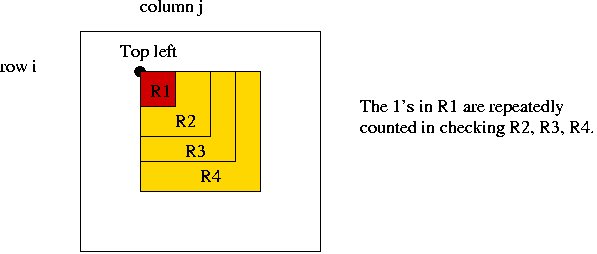=> Small rectangles enclosed by larger ones are always scanned when processing the larger ones.

• Use bottom up approach: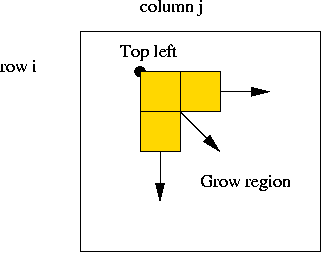• Start at topleft corner (i, j).
• Grow region rightwards and downwards as much as possible.

• A key observation: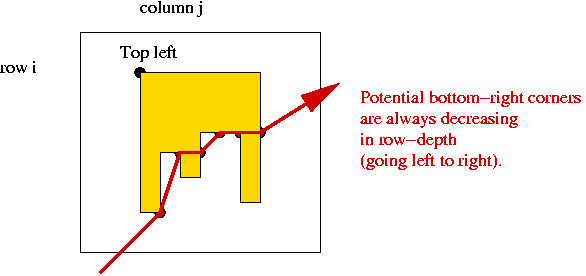• Consider potential bottom-right corners.
• These form an ascending sequence right to left.
=> never need look "deeper" than in previous column.

• Code:
```
// ...

// Start with top left at i,j and find largest rectangle of 1's.
// Use java.awt.Point to store and return two integers.

Point growRegion (int i, int j)
{
// 1. best_a and best_b will record the best bottom-right corner so far.
int best_a = i,  best_b = j;

// 2. a and b will range over possible locations for the bottom-right corner.
int a = i,  b = j;

// 3. There is no need to search below rowMax, which is updated
//    as we proceed.
int rowMax = M-1;

// 4. Scan left to right along row i using index b as long as there are 1's.

while ( (b <= N-1) && (A[i][b]) != 0) {

// 4.1 Start at the highest possible row, row i.
a = i;

// 4.2 Descend into current column (column b) as far down as possible.
while ( (a <= rowMax) && (A[a][b] == 1) )
a = a + 1;

// 4.3 Back up to the last "1".
a = a - 1;

// 4.4 Update rowMax if we stopped at an earlier row.
if (a < rowMax)
rowMax = a;

// 4.5 Check to see if found a larger rectangle.
int area = computeArea (i, j, a, b);

// 4.6 If the rectangle is larger, update.
if (area > maxArea) {
best_a = a;
best_b = b;
maxArea = area;
topLeftX = i;  topLeftY = j;
botRightX = best_a;  botRightY = best_b;
}

// 4.7 Continue with next column.
b++;

} // endwhile

// 5. Return best bottom-right corner.
return new Point (best_a, best_b);
}

public int findMaxRectangleArea (int[][] A)
{
// ...

// 1. Check if array is all zeroes.

// ...

// 2. If all zeroes, no further checks are required.

// 3. We know there's at least one 1 x 1 rectangle (of area 1).
maxArea = 1;

// 4. Outer double-for-loop to consider all possible positions
//    for topleft corner.

for (int i=0; i < M-1; i++) {
for (int j=0; j < N-1; j++) {

// 4.1 Find the largest possible rectangle with topleft at i,j.
Point p = growRegion (i, j);

// NOTE: growRegion itself updates the current largest rectangle,
//       so there's no need to do it here.

}

} // end-outermost-for

// 5. Return value.
return maxArea;
}

// ...

```

• Have we reduced the complexity?
• Potential topleft corners: O(mn).
• Each execution of growRegion is O(mn), worst-case.
=> O(m2 n2) overall.

An improvement:

• As the top-left moves along a row, some columns are repeatedly scanned: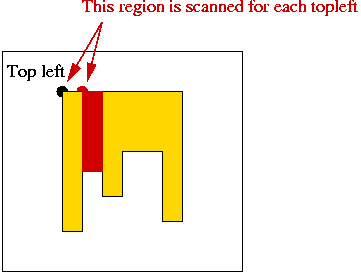• Idea:
• We only need the number of 1's.
=> use a cache.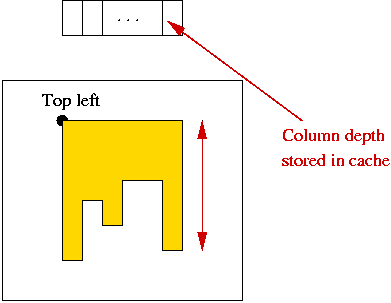• Pre-compute cache for each row before moving topleft-corner along row.

• Code:
```
// ...

// Start with top left at i,j and find largest rectangle of 1's.

Point growRegion (int i, int j)
{
// 1. best_a and best_b will record the best bottom-right corner so far.
int best_a = i,  best_b = j;

// 2. a and b will range over possible locations for the bottom-right corner.
int a = i,  b = j;

// 3. There is no need to search below rowMax, which is updated
//    as we proceed.
int rowMax = M-1;

// 4. Scan left to right along row i using index b as long as there are 1's.

while ( (b <= N-1) && (A[i][b]) != 0) {

// Replace this:
//   a = i;
//   while ( (a <= rowMax) && (A[a][b] == 1) )
//     a = a + 1;
//   a = a - 1;
// with:

// 4.1 Descend into current column (column b) as far down as possible - in time O(1)!
a = i + cache[b] - 1;

// 4.2 Update rowMax if we stopped at an earlier row.
if (a < rowMax)
rowMax = a;
else
a = rowMax;

// 4.3 Check to see if found a larger rectangle.
int area = computeArea (i, j, a, b);

// 4.4 If the rectangle is larger, update.
if (area > maxArea) {

// ...

}

// 4.5 Continue with next column.
b++;

} // endwhile

// 5. Return best bottom-right corner.
return new Point (best_a, best_b);
}

// For each row, create the cache that's used repeatedly in the row.

void fillCache (int i)
{
// 1. Initialize, since cache is created just once.
Arrays.fill (cache, 0);

// 2. Walk across the columns.
for (int j=0; j < N; j++) {

// 2.1 For each column position (i.e., potential top-left corner),
//     find the longest column of 1's.
for (int a=i; a < M; a++) {
if (A[a][j] == 0)
break;
else
cache[j] ++;
}

} // end-column-scan.

}

public int findMaxRectangleArea (int[][] A)
{
// ...

// Create space for cache - use maximum possible size.
cache = new int [N];

// ...

for (int i=0; i < M-1; i++) {

// Fill cache for row i.
fillCache (i);

// Scan columns in row.
for (int j=0; j < N-1; j++) {

// Find the largest possible rectangle with topleft at i,j.
Point p = growRegion (i, j);

}

} // end-outermost-for

// ...

}

// ...
```

• Improvement in complexity:
• We have reduced growRegion to O(n) (number of columns).
• Overall: O(m n2).
• However, we require O(n) additional space.

Note: the material on the maximal rectangle problem is based on an article by D.Vanderwoode in Dr.Dobbs Journal, 1998. Much of the code is completely re-written here (in Java).